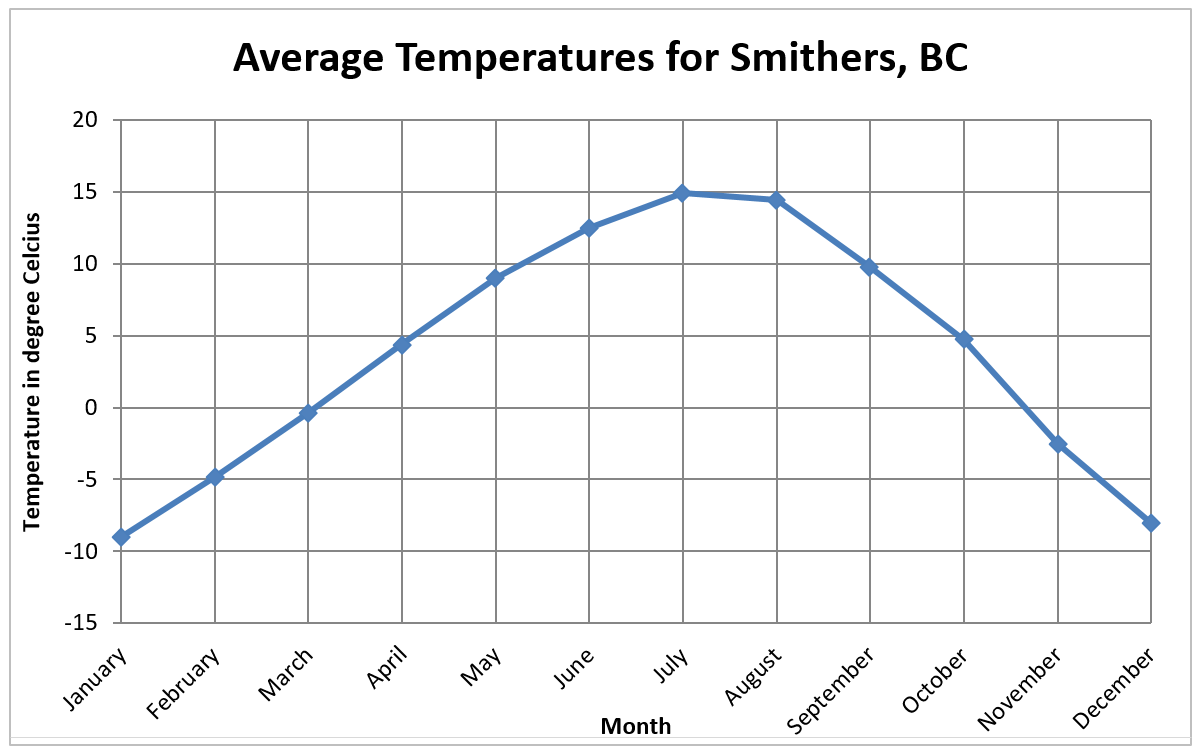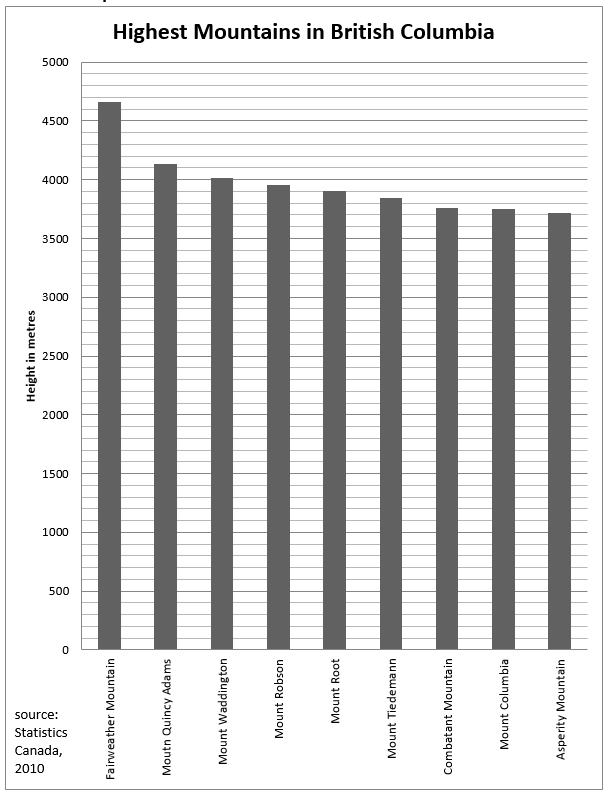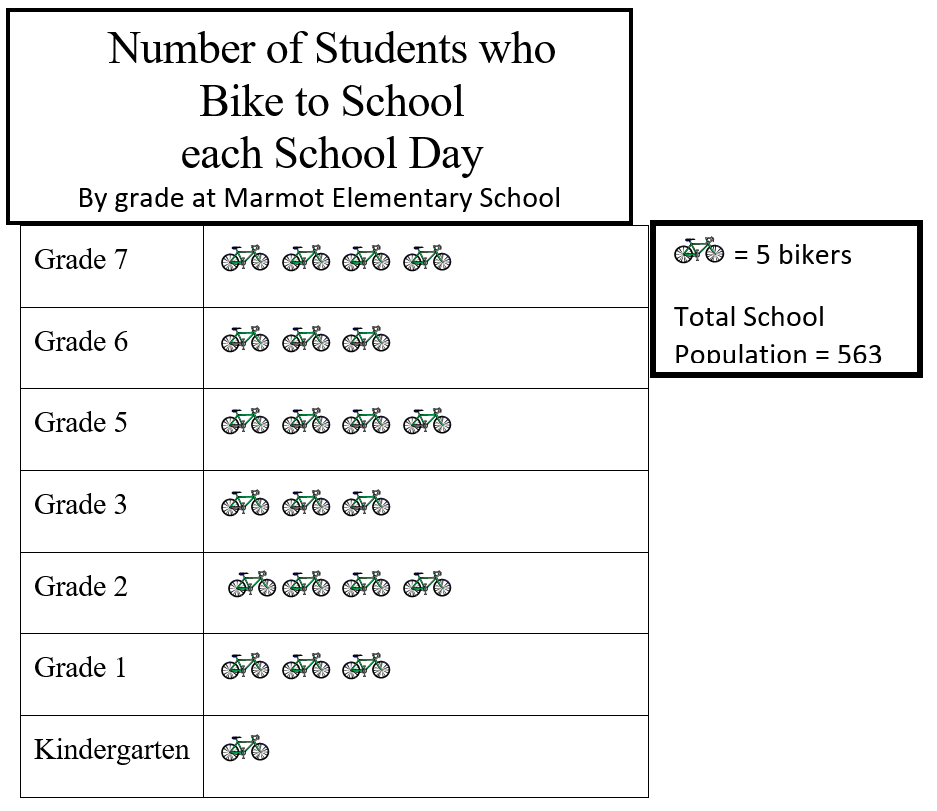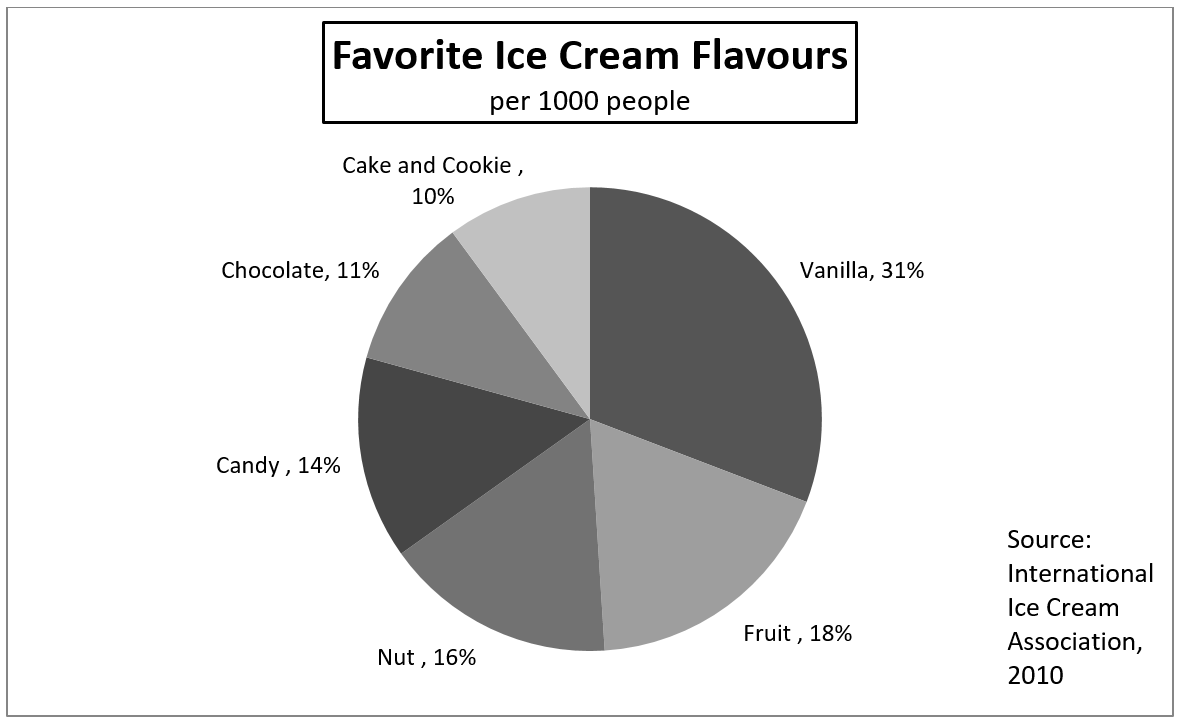# Book 6 Review

You will now practice all the skills you learned in Book 6. You can use this as a review for your final test.

If you can’t remember how to do a question, go back to the lesson on this topic to refresh your memory. The unit and topic for where each question came from is listed next to the question.

Example: 1A means Unit 1, Topic A

# Unit 1

## 1-A

1. Write the ratios asked for.
1. Lillian biked for 6 hours, and covered a total of 45 km. What is the ratio of kilometers to hours?
2. Nine hundred cars were lined up at the ferry terminal. 300 hundred cars got on the next sailing. Write a ratio of how many cars were left behind to how many cars got on the first sailing.

## 1-B

1. Simplify these ratios.
1. $9:12$
2. $50:5$
3. $56:7$
4. $100:120$

2. Write the following ratios as rates.
1. 110 kilometres to 2 hours
2. 9 cups of flour to 3 tablespoons of yeast
3. 240,000 people to 300 square kilometers

## 1-C

1. Solve these proportions.
1. $1:3 = \textit{N}:12$
2. $25:\textit{N} = 20:4$
3. $\textit{N}:49 = 14:98$
4. $4\tfrac{1}{2}:6 = \textit{N}:3.6$
5. The dose for cough syrup is 20 millilitres for each 100 pounds of body weight. How much should be given to a 34 pound child? Round to the nearest millilitre.

# Unit 2

## 2-A

1. Write these percents using numerals and the percent sign.
1. Seventy-two percent
2. Three-fourths percent
3. One hundred two percent

2. Write these percents in words.
1. $12\%$
2. $\tfrac{1}{5}\%$

3. Change the percents to equivalent decimals.
1. $17\%$
2. $98\tfrac{1}{2}\%$
3. $\tfrac{1}{3}\%$

4. Write the decimals as percents.
1. $0.45$
2. $4.75$
3. $0.099$

5. Change each percent to an equivalent common fraction. Put the fraction in lowest terms.
1. $33\tfrac{1}{3}\%$
2. $14\%$
3. $250\%$

6. Write the percent equivalent
1. $\tfrac{1}{5}$
2. $\tfrac{2}{3}$
3. $\tfrac{1}{4}$

# Unit 3

## 3-A

1. Find the answers.(Express percents rounded to the nearest tenth, money to the nearest cent and decimals to the nearest thousandth. Please show all your work. Use proportion.)
1. $13\%\text{ of }52 =$
2. $\tfrac{9}{10}\%\text{ of }2,400$ is                    .
3. What is $135\%\text{ of }1,080$?

2. Solve these problems. Be sure to show all your work.
1. Marianne is renovating her kitchen, and she is ordering everything from her local hardware store. She is getting a sink for $204.79, a dishwasher for$524.95, a counter for $949.99, flooring for$719.95, and a fridge for $579.49. 1. Calculate the HST (12%). 2. Calculate the total cost, including the taxes. 2. Shane sold a home for$340,500.00 for a client. He earned 6% commission. How much money did Shane make?
3. A love seat is originally priced at $904.00, it is offered at 45% off. What is the discount price? 4. Calculate the total cost in Canadian dollars of this purchase made in the United States. Assume$1.00 Canadian = $0.92 U.S. $\text{Clothes} – \text{Total price in }\text{US} = 317.98$. 1. Price in Canadian dollars 2. Duty at 13.5% 3. Total of Canadian value + duty 4. HST (12%) on Canadian value + duty 5. Total cost in Canadian dollars # Unit 4 ## 4-A 1. Find the answers. 1. 34 is what percent of 85? 2. What % of 150 is 114? 3. 33⅓% of what number is 60? 4. 32 is 20% of what number? 5. 75% of what number is 675? 6. 3.75 is 1¼% of? 2. Solve these problems. Be sure to show all your work. 1. A printer is priced at$399. It is marked 30% off.
1. What is the sale price of the printer?
2. What is the cost of this printer with HST (12%)?
2. The Vancouver Fire and Rescue Service has 797 uniformed personnel, about 0.8% are women. About how many women are in uniform in the Vancouver Fire and Rescue Service?
3. Jake is a computer salesperson. He receives a monthly salary of $1,055 plus 15% on all his sales over$5,500. What was his total monthly earnings if his sales were
$12,400 in one month? 4. The local ski hill sold 3,800 season’s passes in 2009. The 2010 sales are down 10.5%. Find the number of season’s passes sold in 2010. # Unit 5 ## 5-A 1. Line graph.[Image Description] 1. Which month has the highest temperature in Smithers? 2. Which month has the lowest temperature in Smithers? 3. Between the months of January to July, is there an increase or decrease in temperature? 4. What is the difference between the monthly temperature for August and the monthly temperature for October? 5. What is the trend of the temperature in Smithers? ## 5-B 1. Bar graph.[Image Description] 1. What is the height of the tallest mountain in BC? 2. How many mountains are over 4,000 metres and under 5,000 metres in height? 3. Which two mountains in this chart are very similar in height? 4. What is the difference (approximately) in height between Fairweather Mountain and Asperity Mountain? ## 5-C 1. Picture graph.[Image Description] 1. How many students in grade 2 ride bikes to school? 2. Which classes have the most bikers? 3. Which class has the least bikers? 4. How many more bikers are in grade 7 than kindergarten? 5. How many students bike in total? 6. What percent of the school bikes each day? ## 5-D 1. Circle graph.[Image Description] 1. Which is the most favourite ice cream flavour? 2. Which is the least liked ice cream flavour? 3. How many people (out of 1,000) like fruit-flavoured ice cream? 4. What percentage of people like vanilla over chocolate? # Answers to Book 6 Review 1. $45:6$ 2. $600:300$ 1. $3:4$ 2. $10:1$ 3. $8:1$ 4. $5:6$ 1. 55 km/hr or $55 \text{km}:1 \text{hr}$ 2. 3 cups of flour to 1 tbsp yeast 3. 800 people to 1 square km 1. $4$ 2. $5$ 3. $7$ 4. $2.7$ 5. $7\text{ mL}$ 1. $72\%$ 2. $\tfrac{3}{4}\%$ 3. $102\%$ 1. Twelve percent 2. One-fifth percent 1. $0.17$ 2. $0.985$ 3. $0.00\overline{3}$ 1. $45\%$ 2. $475\%$ 3. $9.9\%$ 1. $\tfrac{1}{3}$ 2. $\tfrac{7}{50}$ 3. $2\tfrac{1}{2}$ 1. $20\%$ 2. $66.\overline{6}\%$ or $66\tfrac{2}{3}\%$ 3. $25\%$ 1. $6.76$ 2. $21.6$ 3. $1,458$ 1.$357.50
2. $3,336.67 1.$20,430.00
2. $497.20 1.$345.63
2. $46.66 3.$392.29
4. $47.07 5.$439.36
1. $40$
2. $76$
3. $180$
4. $160$
5. $900$
6. $300$
1. $279.30 2.$312.82
1. 6
2. \$2,090
3. 3,401
1. July
2. January
3. Increase
4. Approximately 9 degrees
5. The temperature goes up from January to July, and goes down from July to December
1. Approximately 4,650 metres
2. 3
3. Combatant Mountain and Mount Columbia
4. Approximately 950 metres
1. 20
3. Kindergarten
4. 15
5. 110
6. Approximately 19.5%
1. Vanilla
3. 180
4. 20%

# Image Descriptions

## Graph 1 (Line Graph)

A line graph displays the average temperature in Smithers, BC each month.

• The horizontal axis lists each month of the calendar year.
• The vertical axis is temperature in degrees Celsius, and contains the numbers -15 to 20 in increments of 5.

The line graph data is represented in the following table:

Average Temperatures for Smithers, BC
Month (Horizontal Axis) Temperature in Degrees Celsius (Vertical Axis)
January ~−9
February ~−5
March ~0
April ~5
May ~9
June ~12.5
July ~15
August ~14
September ~10
October ~5
November ~−2.5
December ~−8

## Graph 2 (Bar Graph)

A bar graph displays the height of the highest mountains in British Columbia in metres.

• The horizontal axis lists the following mountains: Fairweather Mountain, Mount Quincy Adams, Mount Waddington, Mount Robson, Mount Root, Mount Tiedemann, Combatant Mountain, Mount Columbia, and Asperity Mountain.
• The vertical axis is height in metres, and contains the numbers 5,000 in increments of 100.

The bar graph data is represented in the following table:

Highest Mountains in British Columbia
Mountain (Horizontal Axis) Height in metres (Vertical Axis)
Fairweather Mountain ~4,650
Mount Robson ~3,950
Mount Root ~3,900
Mount Tiedemann ~3,850
Combatant Mountain ~3,750
Mount Columbia ~3,750
Asperity Mountain ~3,700

## Graph 3 (Picture Graph)

A picture graph displays the number of students at Marmot Elementary School who bike to school each day by grade.

• The horizontal axis contains pictures of bicycles, with each bicycle representing 5 bikers according to the legend.
• The total school population is 563 students.

The picture graph data is represented in the following table:

Number of Students who Bike to School each School Day: By grade at Marmot Elementary School
Grade (Vertical Axis) Pictures of Bikes (Horizontal Axis)
Kindergarten 1

## Graph 4 (Circle Graph)

A circle graph displays the favourite ice cream flavours per 1,000 people.

• The entire graph represents all 1,000 people surveyed for their favourite ice cream flavour.
• Each part represents an ice cream flavour and its popularity as a percent of the whole. The flavours are (clockwise from top): Vanilla, Fruit, Nut, Candy, Chocolate, and Cake and Cookie.

The circle graph data is represented in the following table:

Favourite Ice Cream Flavours: per 1000 people
Flavour Percentage of Total
Vanilla 31%
Fruit 18%
Nut 16%
Candy 14%
Chocolate 11%